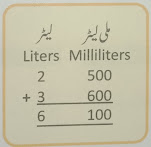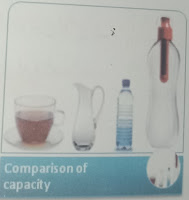# Lesson Planning of Units of Capacity

Lesson Planning of Addition and Subtraction of Units of Capacity

Subject Mathematics

Students` Learning Outcomes

• Add and subtract expressions involving units of capacity / volume.
• Use appropriate unit to measure the capacity / volume of different objects.
• Solve real life problems involving conversions addition and subtraction of units of capacity / volume.

Information for Teachers

• There are two standard units of capacity / volume that are liter (L), and millimetres (ML)
• If liters & millilitres appear in mixed form in any expression then we write liters & millilitres separately below the headings of liter and millilitre.
• After writing the data below the columns we add or subtract.
• Liter can be converted into millilitres by multiplying liters with 1000 so, ml = l x 1000 ml.• Milliners can be converted into liters by dividing with 1000 so.
• During this lesson consult at each level = ml/1000 where it needed.

Material / Resources

Writing board, chalk/marker, duster, flashcards, empty bottles of different capacities, bucket, cup, jug, syringe and textbook

Introduction

• Ask the students when you go to petrol pump, in which “units” petrol is filled? (Expected answer would be as; liters)
• Tell them that we make columns of liters and millilitres separately and below each column we write number of liters or millilitres and then add or subtract same units.
• Recall the methods of conversion of units.
• If we want to convert greater unit in small then we use multiplication and use division if we want to convert smaller unit in greater one.

Development

 Activity 1 State the following situations and solve it with their help on the board, as;  If gallon “A” holds 2 l and 500 ml of milk, gallon “B” holds 3 l and 600 ml of milk then what will be the total quantity of milk? Ask the students what we will use addition or subtraction?Milk of gallon A = 2 l and 500 ml Milk of gallon B = 3 l and 600 ml Total = ____?   As 500 + 600 equals to 1100 and 1 liter = 1000 ml so it is 100 ml more than 1 liter. Therefore, 1 liter will be added to liter column and extra 100 ml will be written in the millilitre column. Examples: Ali collected 5 l and 500 ml rain water in a bucket. He used 3 liters and 250 ml to water his plants. How much water is left? Ask the students what would we use addition or subtraction for solving this problems?(Expected answer would be as; subtraction) Solve this problem of the board: as; Bucket contains water = 5 l and 500 mlWater used = 3 l and 250 ml Remaining water =  ____?

 Activity 2 Display a cup, jug, small and large bottle of different capacities. Call one student and ask him to arrange the items from smaller to greatest capacity.Ask the students how do you know this is the correct order? Tell them that you can observe the size of each object. Their size represents their capacity of holding the liquid.

 Activity 3 Assign these types of products to the students and ask them to write the capacities of the following in liters or millilitres and then convert them in greater or smaller units:-Sum up / Conclusion
• If we want to convert greater unit in small then we use multiplication and use division if we want to convert smaller unit in greater one.
• Only same units can be added or subtracted if liters & millilitres appear in mixed from in any expression then we write liters & millilitres separately below the heading of liter and millilitre.

Assessment

• Ask them to solve the following questions in their notebooks: as;
• Check their work and guide them if they feel any problem.